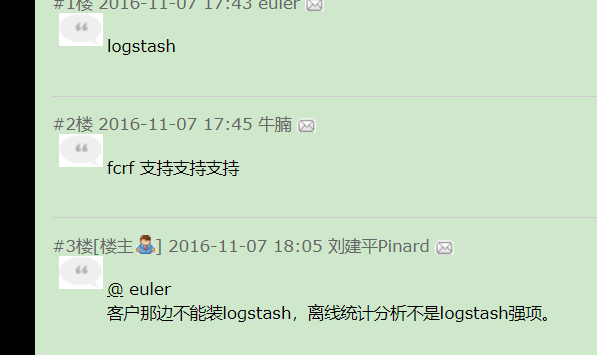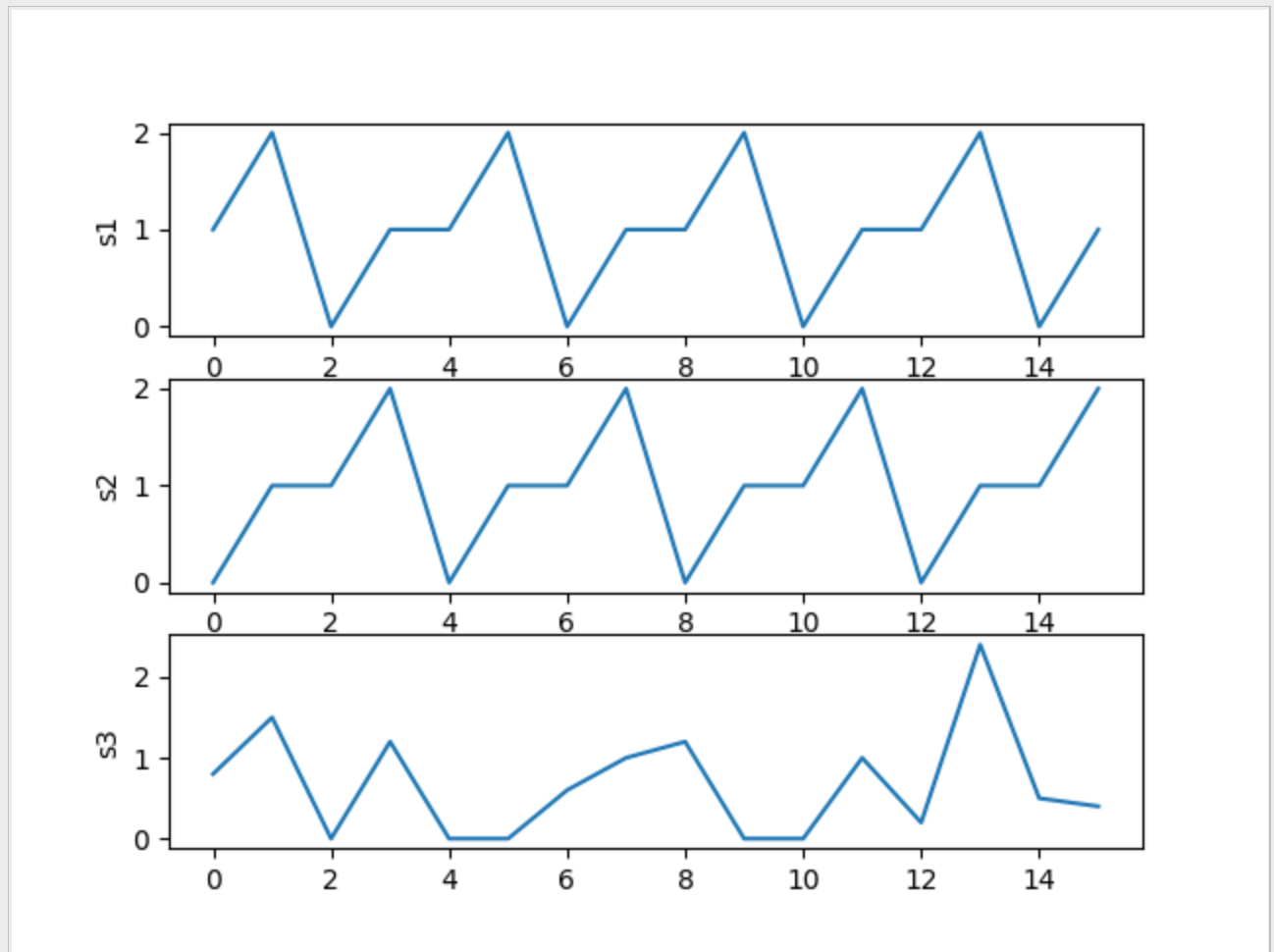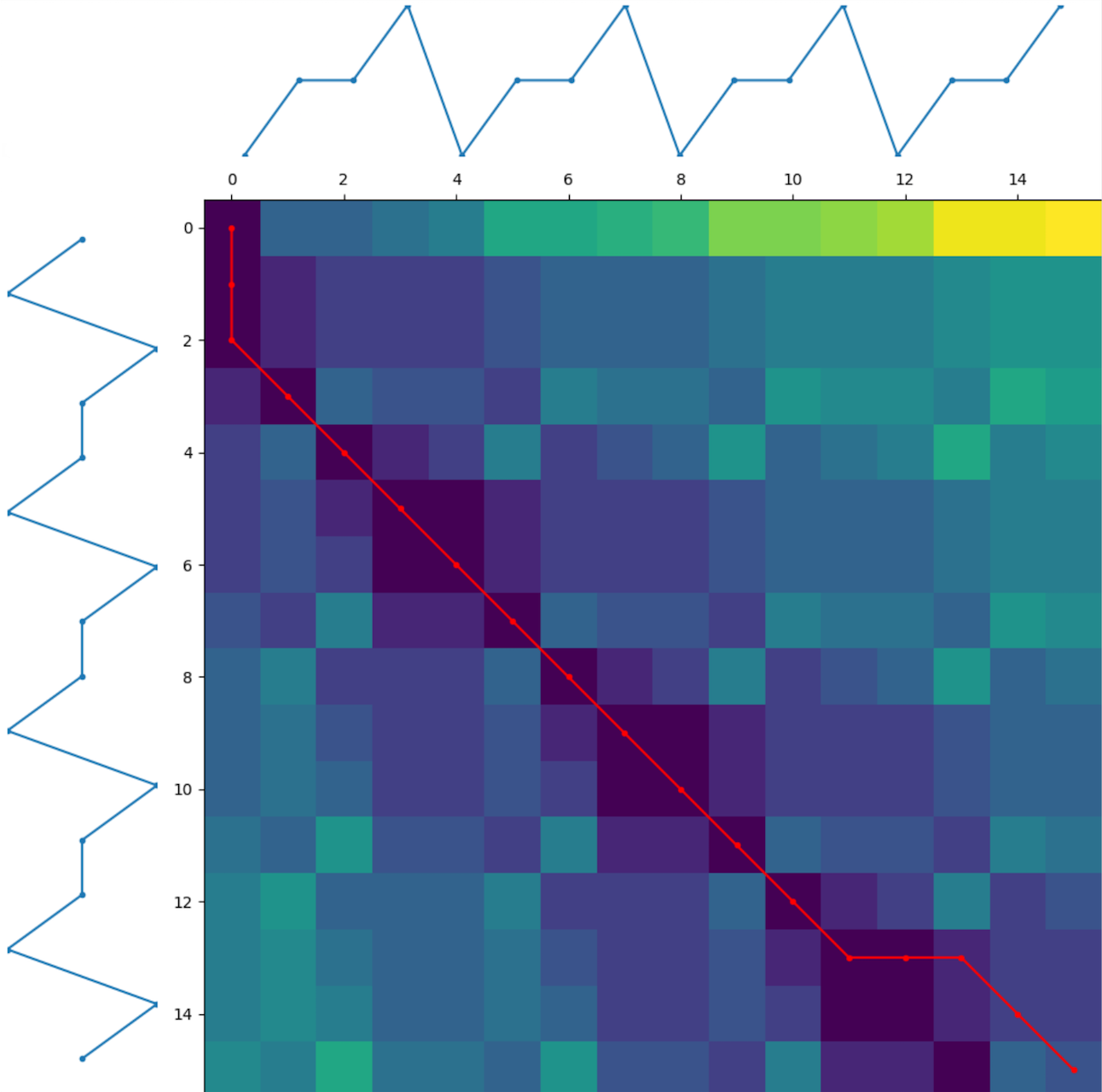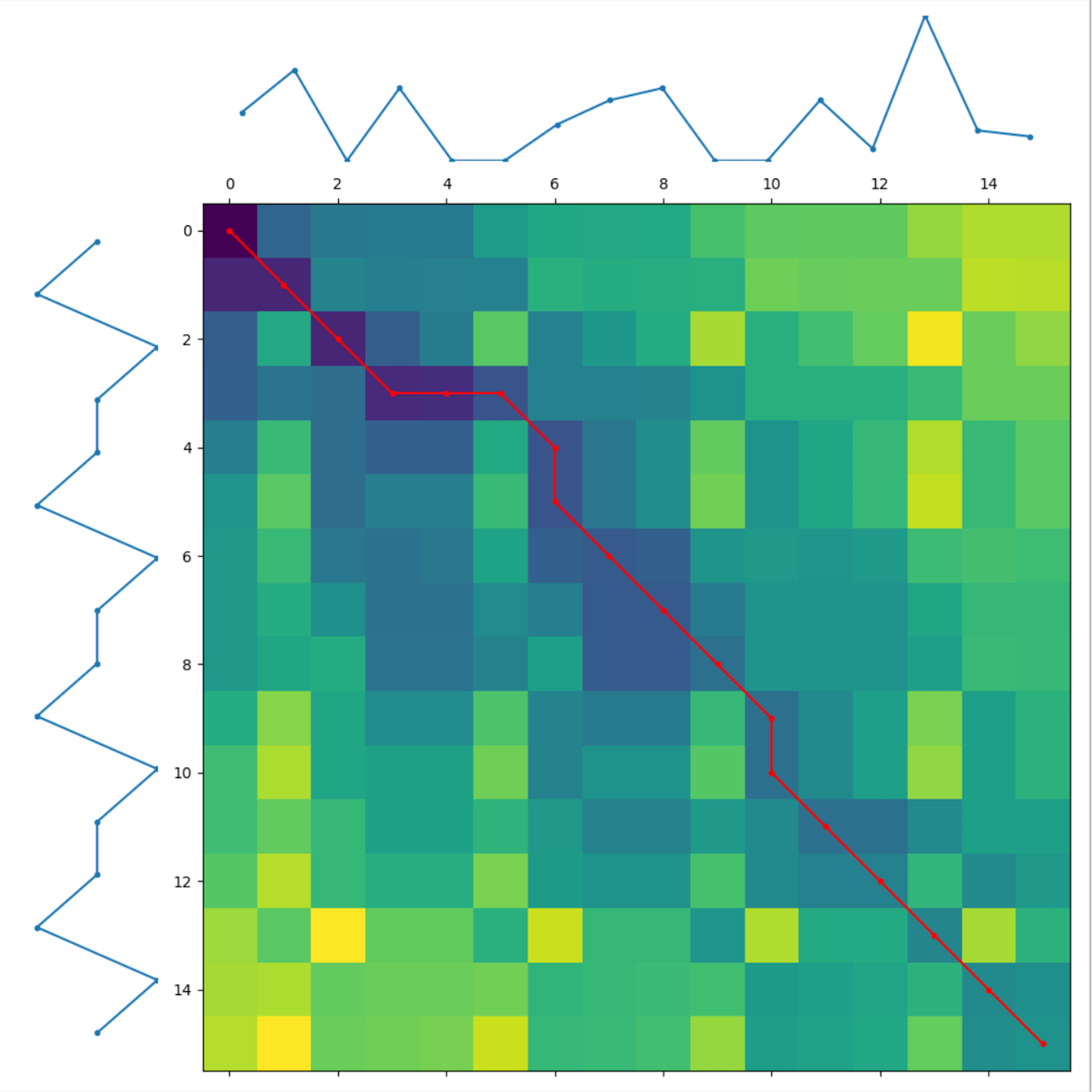• 为此,主要针对多元时间序列的相似性搜索进行综述,归纳了主要的相似模式度量方法,对比了不同相似模式度量下的序列搜索方法,并分析了不同方法的优缺点,以期为进一步研究多元时间序列相似性搜索提供帮助.
• 现有的多元时间序列相似性度量方法难以平衡度量准确性和计算效率之间的矛盾。针对该问题，首先，对多元时间序列进行多维分段拟合；然后，选取各分段上序列点的均值作为特征；最后，以特征序列作为输入，利用动态时间...
• 目前，时间序列相似性大多是在原始序列上进行判断和比较的，原始序列维度较高，计算量大，不利于相似性比较。提出了新的关键点（转折点或极值点）算法，除利用常用的极值法求非单调序列的关键点外，还提出了求单调...
• 针对欧几里德距离描述分段趋势的不足和各种模式距离对应分段之间距离值的离散化问题，提出一种基于形态相似距离的时间序列相似性度量方法，标准数据集上完成的识别和聚类实验表明了该方法的可行性和有效性。
• 针对传统符号聚合近似方法在特征表示时容易忽略时间序列局部形态特征的局限性,以及动态时间弯曲在度量上的优势,提出一种基于数值符号和形态特征的时间序列相似性度量方法.将时间序列进行符号和形态的特征表示后,提出...
• 为了提高时间序列子序列匹配的准确度和效率，提出了基于极值点特征的时间序列相似性查询方法。首先识别出时间序列中的极值特征点，根据极值点使用多层次极值划分法对长序列进行划分；然后对划分得到的多层次子序列集...时间序列 数据挖掘
• 时间序列相似性度量在挖掘时间序列模式，提取时间序列关联关系上发挥着重要作用。分析了当前主流的时间序列相似性度量算法，分别指出了各度量算法在度量时序数据相似性时存在的缺陷，并提出了基于数学形态学的时间...
• 由于时间序列的长度很大，并且不确定时间序列在每个采样点的取值具有不确定性，导致时间序列在相似性匹配和聚类挖掘中时间复杂度很高，为了解决该问题，提出了基于趋势的时间序列相似性度量方法和聚类方法。...时间序列 不确定性 相似性度量 聚类
• 摘要：针对数据挖掘领域中时间序列相似性度量问题，提出了基于斜率偏离度量的浮动索引相似性搜索算法。在斜率偏离度量的基础上建立分箱标志，通过浮动索引方法有效实现高维多元时间序列的准确索引，用实际的飞行...时间序列 相似性搜索
• 计及数据分布特性与形态波动特征，改进时间序列相似性度量方法，将欧氏距离与改进后的动态时间弯曲距离相结合求取负荷曲线和新能源出力曲线的相似性距离，将其定义为源荷相似性距离作为新能源-负荷特性指标。...
• 现有的各种多元时间序列相似性搜索方法难以准确高效地完成搜索任务。提出了一种基于特征点分段的多元时间序列相似性搜索算法，提取所定义的用于分段的特征点，分段后将原时间序列转化为模式序列，该模式序列能够很好...
• https://blog.csdn.net/key_v/article/details/47733319 https://www.cnblogs.com/pinard/p/6039099.html https://github.com/stanford-futuredata/FAST

https://blog.csdn.net/key_v/article/details/47733319
https://www.cnblogs.com/pinard/p/6039099.htmlhttps://github.com/stanford-futuredata/FAST

展开全文• 时间序列相似性度量领域中，现有的算法对各类相似性变形的识别能力有限。为了能有效支持识别多种相似性形变，提出涨落模式（FP）的概念，以涨落模式保存原序列的趋势变化信息，利用最长公共子序列算法计算涨落模式的...时间序列 相似性度量 分类
• 时间序列相似性度量综述

千次阅读 2021-02-03 03:53:08
时间序列相似性属于曲线相似性/曲线匹配(curve matching)领域的内容，在这一领域，有许多有用的方法，但是国内的博客上鲜有这方面的内容，因此我选取了几种常用的方法进行一下综述性的阐述。衡量相似性之前，我们...

时间序列相似性属于曲线相似性/曲线匹配(curve matching)领域的内容，在这一领域，有许多有用的方法，但是国内的博客上鲜有这方面的内容，因此我选取了几种常用的方法进行一下综述性的阐述。

衡量相似性之前，我们首先定义“相似”。

正常情况下，我们认为x,y,z是形状相似的，在这三条曲线中，我们认为y，z是最相似的两条曲线(因为y,z的距离最近)。

ok，那我们先来看看寻常意义上的相似：距离最近且形状相似。本文主要详细介绍时间序列相似度计算的DTW算法和PLR算法。

1. 欧式距离

要衡量距离与形状，显然欧式距离是一个天然完美的指标，上图中我们正是基于欧式距离认为y与z是最相似的，欧式距离在诸多算法都有广泛的应用。对于长度相同的序列，计算每两点之间的距离然后求和，距离越小相似度越高(whole matching)。对于不同长度的序列，一般有两种方法处理：

1)子序列匹配(subsequence matching): 找出长序列中与短序列最相似的部分。举个栗子，设序列,序列，其中。滚动地计算A与B的距离：，然后找出所有d中的最小值，该距离所对应的A序列的索引即为A中与B最相似的部分。

2)滑动窗口：微软在2001年在Dimensionality Reduction for Fast Similarity Search文中提出为了减少算法复杂度，可以复制B序直到与A序列等长。

由于微软之后使用了独特的降维方法，且计算复杂度不是本文考虑的主要内容，因此，在涉及长短序列相似度计算的时候，本文均使用第一种方法。

似乎时间序列的相似性度量的计算可以就此为止了，然而远非如此。

天津大学的XIAO-LI DONG, CHENG-KUI GU, ZHENG-OU WANG在2006年Research on shape-based time series similarity measure[C]//2006 International Conference on Machine Learning and Cybernetics. IEEE, 2006: 1253-1258一文中指出了欧式距离用于衡量时间序列相似性的三个缺陷：不能辨别形状相似性

不能反映趋势动态变化幅度的相似性

基于点距离的计算不能反映不同分析频率的不同

举个栗子：

A与B的变化趋势几乎完全相反，A与C的变化趋势几乎完全相同。如果使用欧式距离去度量，那么结论就是A与B是最相似的。而实际上，在变化是A与C是相似的。

为了进一步加强对欧式距离的理解，我们不妨再举一个简单的例子：

正常来说，我们认为与y1最相似的是y3，实际上，y3就是y1向下平移得到的。然而欧式距离告诉我们，距离y1最近的是y2。

下面是使用Python进行模拟的源代码：

import numpy as np

import matplotlib.pyplot as plt

x=np.arange(0,np.pi*2,0.1)

y1=np.sin(x)

y2=np.cos(x)-2

y3=y1-2

plt.plot(y1)

plt.plot(y2)

plt.plot(y3)

plt.legend(['y1','y2','y3'])

def dis(x,y):

return(sum(x-y)**2)

dis(y1,y2)

Out: 15831.914509398732

dis(y1,y3)

Out: 15876.0

欧式距离对形状的度量如此糟糕，有没有更好的模型能度量形状呢？

2. 模式距离Pattern distance

首先引入一个算法，PLR(piecewise linear representation)分段线性表示。

一个时间序列，无非有三种状态：上升、下降和不变，我们将这种状态对应表示为。假设有某个长度为S的序列，我们将其等分为K段。对于每一段计算一个斜率，斜率为正表示上升，为负表示下降，为0表示不变。

我画了一个草图，可能emm……不是很美观。

那么我们就可以将这个序列表示为[1,1,0,-1...]这样的序列，将相邻的相同模式进行合并，我们得到[1,0,-1...]的序列。这就是PLR算法。

关于PLR算法的点的分割，为了便于说理，我这里直接用的是等分的方式，然而观察上图可知，第三个模式表示为了0，实际上第三个模式是一个尖峰，是先上升后下降的，所以等分切割的方法并不科学。

KEOGH E. 在Fast similarity search in the presence of longitudinal scaling in time series data bases中提出的自底向上的搜索方法很好的解决了这个问题，感兴趣的读者可以自行了解。

因为我们将相邻的相同模式进行了合并，所以我们得到的模式序列一定是1,-1,0间隔排列的，每一个模式可能跨越了不同的时间长度，在合并模式后，序列S1可能有N个模式，S2可能有M个模式。现在我们要将他们等模式数化。

如上图所示，经过PLR后，我们将S1，S2表示为：分表表示S1,S2的第i,j个模式，即，t表示时间，且无论怎么切割，最后的终点都是相等的，即。结合上图，具体一点就是：等模式数化后将他们变形为：说白了就让他们使用共同的分割点，以获得最后长度相等的模式序列。

OK现在我们已经完全定义好了何为模式，接下来我们计算距离。这里我们使用绝对值距离，当然使用欧式距离也是可以的。模式距离公式为：，显然，距离越靠近0，表示模式越相似；越靠近2，表明模式越不相似。把所有的模式距离加起来即时间序列的模式距离：嗯……目前为止看起来还不错。但是需要注意的是，每一个模式可能跨越了不同的时间长度，而一个模式持续时间越长，它包含整个序列的信息就越多，这启发我们需要进行加权。因此：，其中为第i个模式所跨越的时间长度，为总时间长度。

3. 形状距离shape distance

在模式距离的基础上，XIAO-LI DONG, CHENG-KUI GU, ZHENG-OU WANG提出了形状距离，进一步提升了度量效果。该算法于2006年在国际机器学习与控制会议上提出。

如果理解了pattern distance的计算，那么理解shape distance将会非常简单，因为shape distance归根到底、总而言之就是加了一个振幅的改变量并重新设定了模式序列。

假设我们已经得到了等模式数化后的序列：设振幅amplitude改变量序列为A，则：，就是我们每一个分割区间的端点对应的序列值值差，那么我们可以得到：形状距离的最终计算公式为：，同样为时间权重。注意原序列S有N个点，模式序列和振幅改变量序列都是只有N-1个点。而这里的模式m也要重新定义。

下面以股票为例进行说明。我们认为股票的价格走势通常有七种状态：{加速下降，水平下降，减速下降，不变，减速上升，水平上升，加速上升}，我们用模式来描述这一点。

现在我们设定一个阈值th来帮助我们区分这7种状态，设Ki表示通过PLR划分后的第K段直线的斜率，还记得在模式距离中怎么设定模式的吗？

如果斜率小于0，则表示下降，记为-1；斜率大于0，则表示上升，记为1；如果斜率等于0，则表示不变，那么记为0。

现在情况稍微复杂了一些，因为我们引入了更多的模式(7种)。此时属于{-3，-2，-1}中的一种，那如何来知晓这支股票是加速下降、水平下降还是减速下降呢？斜率的改变量可以帮助我们，如果下一期斜率的改变量小于0，那说明斜率在递减，直线变得更陡峭了，是加速下降的，因此设为-3模式。如果，那么是水平下降的，设为-2。如果,说明是减速下降的，设为-1。如果斜率的在这个范围内，那么就认为是近似不变的，设为0。以此类推。

用一张表来总结一下：

形状距离公式是满足以下四个距离定理的：

此外，为了提高模型的准确度，较少绝对值的差异对整个模型的影响，一般需要对原序列进行标准化处理。完整的算法流程图如下：

形状距离由于加入了更多的模式，使得距离的度量更加精确，效果会好于模式距离。

4. DTW

讲了这么多距离，有没有更简单的方法来度量时间序列的相似性呢？相关系数可以吗？

相关系数是一个非常优美的指标，不仅能衡量相关性，也能衡量相似性，但是在时间序列中一般不使用相关系数衡量相似性，这是因为它不能解决图形平移的问题。

还是举个栗子~

y1与y2是平移的关系，显然与y1最相似的应该是y2，然而相关系数告诉我们是y3。

下面是Python进行模拟的代码：

import numpy as np

x=np.arange(-np.pi*2,np.pi*2,0.1)

y1=np.sin(x)

y2=np.cos(x)

y3=x

plt.plot(y1)

plt.plot(y2)

plt.plot(y3)

plt.legend(['y1','y2','y3'])

np.corrcoef([y1,y2,y3])

Out:

array([[ 1.00000000e+00, -1.76804105e-04, -3.88194383e-01],

[-1.76804105e-04, 1.00000000e+00, -1.28528471e-02],

[-3.88194383e-01, -1.28528471e-02, 1.00000000e+00]])

DTW(dynamic time warping)动态时间规整就是专为解决此问题而生~

DTW实际上是计算欧式距离，我们在之前讲过，欧式距离不能很好地度量形状相似性。左边这幅图更清楚地表现了这一点，按照一般欧式距离的计算方法，所有点直接对应下来。而DTW就是找到序列之间正确对应的点再计算他们的距离。而由于DTW这一出色的特质，这使得DTW在语音识别领域有着广泛的应用。因为一个音节，它可能拖的很长或很短，如何去正确地识别相似声音或音节对于语音识别至关重要。图源自：https://blog.csdn.net/zouxy09/article/details/9140207

如上图所示，a对应的点应该是b而不是b'，DTW的根本任务就是将点进行正确的对应。

那正确对应的标准是什么呢？

DTW认为，如果两个序列的点正确对应了，那么他们的距离(欧式距离)达到最小。

OK，现在问题很简单了，就找距离最小的点。一个序列的一个点可能对应另一个序列的多个点，如果穷举出所有的可能去找出最合适的点，这在时间复杂度上属于一个NP难的问题。我们需要一个行之有效的算法——动态规划。

由于DTW的理论比较晦涩，直接看公式让人云里雾里，为了更清楚简单地把DTW讲明白，我用Python进行一遍模拟的计算。

简单点，说话的方式简单点~~~

import pandas as pd

import numpy as np

import matplotlib.pyplot as plt

import seaborn as sns

x = np.array([1, 1, 2, 3, 2, 0])

y = np.array([0, 1, 1, 2, 3, 2, 1])

plt.plot(x,'r', label='x')

plt.plot(y, 'g', label='y')

plt.legend()

生成了两个长度不等的序列x,y。

distances = np.zeros((len(y), len(x)))

for i in range(len(y)):

for j in range(len(x)):

distances[i,j] = (x[j]-y[i])**2

distances

Out:

array([[1., 1., 4., 9., 4., 0.],

[0., 0., 1., 4., 1., 1.],

[0., 0., 1., 4., 1., 1.],

[1., 1., 0., 1., 0., 4.],

[4., 4., 1., 0., 1., 9.],

[1., 1., 0., 1., 0., 4.],

[0., 0., 1., 4., 1., 1.]])

计算两个序列的距离矩阵。横着表示x序列，竖着是y序列，比如说第0行第0个元素1表示x序列的第0个值和y序列的第0个值的距离(Python的索引从0开始)，即;再比如第4行第1列的元素4(如果你的第4行第1列元素没有找到4的话，请把索引从0开始)表示x序列第1个值和y序列的第4个值间的距离，即我们来做个可视化，颜色越深表示距离越远，比如说最远的距离是x的第三个值和y的第0个值之间的距离。

这里注意一下，我们的图形和距离矩阵是没有一一对应的。我们的距离矩阵中，索引0从左上角开始，图形从左下角开始。

def distance_cost_plot(distances):

plt.imshow(distances, interpolation='nearest', cmap='Reds')

plt.gca().invert_yaxis()#倒转y轴，让它与x轴的都从左下角开始

plt.xlabel("X")

plt.ylabel("Y")

# plt.grid()

plt.colorbar()

distance_cost_plot(distances)

现在我们计算一个累积距离矩阵，累积距离最小是我们的最终目标。

accumulated_cost = np.zeros((len(y), len(x)))

accumulated_cost[0,0] = distances[0,0]

accumulated_cost

Out:

array([[1., 0., 0., 0., 0., 0.],

[0., 0., 0., 0., 0., 0.],

[0., 0., 0., 0., 0., 0.],

[0., 0., 0., 0., 0., 0.],

[0., 0., 0., 0., 0., 0.],

[0., 0., 0., 0., 0., 0.],

[0., 0., 0., 0., 0., 0.]])

distance_cost_plot(accumulated_cost)

显然累积距离矩阵的第0行第0列=距离矩阵的第0行第0列=1，我们必须经过起点吧……如果我们一直往右走，那么累积距离距离矩阵：

for i in range(1, len(x)):

accumulated_cost[0,i] = distances[0,i] + accumulated_cost[0, i-1]

accumulated_cost

array([[ 1., 2., 6., 15., 19., 19.],

[ 0., 0., 0., 0., 0., 0.],

[ 0., 0., 0., 0., 0., 0.],

[ 0., 0., 0., 0., 0., 0.],

[ 0., 0., 0., 0., 0., 0.],

[ 0., 0., 0., 0., 0., 0.],

[ 0., 0., 0., 0., 0., 0.]])

distance_cost_plot(accumulated_cost)

如果我们一直往上走，那么：

for i in range(1, len(y)):

accumulated_cost[i,0] = distances[i, 0] + accumulated_cost[i-1, 0]

accumulated_cost

array([[ 1., 2., 6., 15., 19., 19.],

[ 1., 0., 0., 0., 0., 0.],

[ 1., 0., 0., 0., 0., 0.],

[ 2., 0., 0., 0., 0., 0.],

[ 6., 0., 0., 0., 0., 0.],

[ 7., 0., 0., 0., 0., 0.],

[ 7., 0., 0., 0., 0., 0.]])

distance_cost_plot(accumulated_cost)

好了，累积距离矩阵的计算方式已经完全明白了。在继续下去之前，必须讲明累积距离矩阵中路径的意义。我们的目标是找一条路径使得累积距离最小。从(0,0)开始，经过这个方块表示将x,y的第0个点对应起来，当然，起点是必须经过的~

如果路径经过(1,0)，表示将x的第1个点和y序列的第0个点对应起来。

如果路径经过(1,1)，表示将x的第1个点和y序列的第1个点对应起来。

所以，路径的前进方向只有三个：向右，向上，斜右上。

这是显然，我们的路径不能后退，如果路径向右或向上了多个方块，这表明一个序列的一个点对应了另一个序列的多个点。

目前为止，我们已经完成了累积距离矩阵的两列的计算。对于任何其他的点，累积距离的新增只可能来自于三个方向：左边，下边，斜左下。所谓动态规划就是我每前进一步都选取使我当前行进距离最小的方向。因此，对于任何其他的点，累积距离的计算公式为：现在，我们把累积距离矩阵计算完整：

for i in range(1, len(y)):

for j in range(1, len(x)):

accumulated_cost[i, j] = min(accumulated_cost[i-1, j-1], accumulated_cost[i-1, j], accumulated_cost[i, j-1]) + distances[i, j]

accumulated_cost

Out:

array([[ 1., 2., 6., 15., 19., 19.],

[ 1., 1., 2., 6., 7., 8.],

[ 1., 1., 2., 6., 7., 8.],

[ 2., 2., 1., 2., 2., 6.],

[ 6., 6., 2., 1., 2., 11.],

[ 7., 7., 2., 2., 1., 5.],

[ 7., 7., 3., 6., 2., 2.]])

distance_cost_plot(accumulated_cost)

现在，最佳路径已经清晰地显示在了累积距离矩阵之中，就是图中颜色最淡的方块。

现在，我们只需要通过回溯的方法找回最佳路径就可以了：

path = [[len(x)-1, len(y)-1]]

i = len(y)-1

j = len(x)-1

while i>0 and j>0:

if i==0:

j = j - 1

elif j==0:

i = i - 1

else:

if accumulated_cost[i-1, j] == min(accumulated_cost[i-1, j-1], accumulated_cost[i-1, j], accumulated_cost[i, j-1]):

i = i - 1#来自于左边

elif accumulated_cost[i, j-1] == min(accumulated_cost[i-1, j-1], accumulated_cost[i-1, j], accumulated_cost[i, j-1]):

j = j-1#来自于下边

else:

i = i - 1#来自于左下边

j= j- 1

path.append([j, i])

path.append([0,0])

path

Out: [[5, 6], [4, 5], [3, 4], [2, 3], [1, 2], [1, 1], [0, 1], [0, 0]]

path_x = [point for point in path]

path_y = [point for point in path]

distance_cost_plot(accumulated_cost)

plt.plot(path_x, path_y)

在刚才，我们已经讲明，最优路径所经过的地方表示两个序列应该对应的点。

计算这些点的欧式距离作为相似性的度量，这就是DTW。

Reference:Dong X L, Gu C K, Wang Z O. Research on shape-based time series similarity measure[C]//2006 International Conference on Machine Learning and Cybernetics. IEEE, 2006: 1253-1258.

Wang D, Rong G. Pattern distance of time series[J]. Zhejiang Daxue Xuebao(Gongxue Ban)/Journal of Zhejiang University(Engineering Science), 2004, 38(7): 795-798.

Kim S, Lee H, Ko H, et al. Pattern Matching Trading System Based on the Dynamic Time Warping Algorithm[J]. Sustainability, 2018, 10(12): 4641.

Keogh E, Chakrabarti K, Pazzani M, et al. Dimensionality reduction for fast similarity search in large time series databases[J]. Knowledge and information Systems, 2001, 3(3): 263-286.

Mori U, Mendiburu A, Lozano J A. Distance measures for time series in R: The TSdist package[J]. R journal, 2016, 8(2): 451-459.

展开全文• 时间序列相似性度量-DTW

万次阅读 多人点赞 2019-01-25 16:44:59
最近项目中遇到求解时间序列相似性问题，这里序列也可以看成向量。在传统算法中，可以用余弦相似度和pearson相关系数来描述两个序列的相似度。但是时间序列比较特殊，可能存在两个问题： 两段时间序列长度不同。...

1. 背景

最近项目中遇到求解时间序列相似性问题，这里序列也可以看成向量。在传统算法中，可以用余弦相似度和pearson相关系数来描述两个序列的相似度。但是时间序列比较特殊，可能存在两个问题：

1. 两段时间序列长度不同。如何求相似度？
2. 一个序列是另一个序列平移之后得到的。如何求相似距离？

第一个问题，导致了根本不能用余弦相似度和pearson相关系数来求解相似。第二个问题，导致了也不能基于欧式距离这样的算法，来求解相似距离。比如下面两个长度不同的序列：

s1 = [1, 2, 0, 1, 1, 2]
s2 = [1, 0, 1]

本文记录一种算法，一方面：如果两个序列中的其中一个序列是另一个序列的平移序列，则可以比较合理的求出两个序列的相似距离。另一方面：也可以求解长度不同序列的相似距离。主要参考：dtaidistance 这个项目的源码和其中的Dynamic Time Warping (DTW)思想。

同时基于这个算法，先计算相似距离，再把相似距离通过 1 1 + d i s t \frac{1}{1+dist} 转化为相似度。这样就可以得到长度不同向量的相似度。

2. 核心思想

Dynamic Time Warping (DTW) 本质上和通过动态规划来计算这个序列的相似距离。其实这和求解字符串的最长公共子串、子序列这类问题本质比较类似，参考：

这里基于动态规划构建序列a和序列b的距离矩阵dp[i][j]，其中dp[i][j]表示序列a[0:i]和b[0:j]之间的相似距离的平方。并且有：
d p [ i ] [ j ] = { ( a [ 0 ] − b [ 0 ] ) 2 i = 0, j = 0 ( a [ 0 ] − b [ j ] ) 2 + d p [ 0 ] [ j − 1 ] i = 0 ( a [ i ] − b [ 0 ] ) 2 + d p [ i − 1 ] [ 0 ] j = 0 ( a [ i ] − b [ j ] ) 2 + m i n ( d p [ i − 1 ] [ j ] , d p [ j − 1 ] [ i ] , d p [ i − 1 ] [ j − 1 ] ) i , j > 0 dp[i][j] = \begin{cases} (a - b)^2 \quad\quad &\text{i = 0, j = 0} \\ (a - b[j])^2 + dp[j-1] \quad\quad &\text{i = 0} \\ (a[i] - b)^2 + dp[i-1] \quad\quad &\text{j = 0} \\ (a[i] - b[j])^2 + min(dp[i-1][j],dp[j-1][i],dp[i-1][j-1]) \quad\quad &i,j > 0 \end{cases}

所以dp[len(a)-1][len(b)-1] 就是相似距离的平方，最后开方就是两个时间序列的相似距离，这也就是DTW算法核心实现。实际上，上文的那个github核心代码其实也就是这么写的，只不过在此基础上加了其他参数、画图、层次聚类的代码实现而已。

3. 实现

在实际使用中解读了dtaidistance这个项目的源码，其中除去所有参数后，DTW最简单的实现如下：

import numpy as np

float_formatter = lambda x: "%.2f" % x
np.set_printoptions(formatter={'float_kind': float_formatter})

def TimeSeriesSimilarity(s1, s2):
l1 = len(s1)
l2 = len(s2)
paths = np.full((l1 + 1, l2 + 1), np.inf)  # 全部赋予无穷大
paths[0, 0] = 0
for i in range(l1):
for j in range(l2):
d = s1[i] - s2[j]
cost = d ** 2
paths[i + 1, j + 1] = cost + min(paths[i, j + 1], paths[i + 1, j], paths[i, j])

paths = np.sqrt(paths)
s = paths[l1, l2]
return s, paths.T

if __name__ == '__main__':
s1 = [1, 2, 0, 1, 1, 2]
s2 = [1, 0, 1]

distance, paths = TimeSeriesSimilarity(s1, s2)
print(paths)
print("=" * 40)
print(distance)

输出

[[0.00 inf inf inf inf inf inf]
[inf 0.00 1.00 1.41 1.41 1.41 1.73]
[inf 1.00 2.00 1.00 1.41 1.73 2.45]
[inf 1.00 1.41 1.41 1.00 1.00 1.41]]
========================================
1.4142135623730951

这里的矩阵
[ 0.00 1.00 1.41 1.41 1.41 1.73 1.00 2.00 1.00 1.41 1.73 2.45 1.00 1.41 1.41 1.00 1.00 1.41 ] \begin{bmatrix} 0.00 & 1.00 & 1.41 & 1.41 & 1.41 & 1.73 \\ 1.00 & 2.00 & 1.00 & 1.41 & 1.73 & 2.45 \\ 1.00 & 1.41 & 1.41 & 1.00 & 1.00 & 1.41 \end{bmatrix}

就是dp[i][j]。这里代码用另一种写法实现了求解dp[i][j]。并且矩阵右下角元素1.41，就是我们求解的相似距离。

4. 改进

其实在实际使用中，我们发现该算法对周期序列的距离计算不是很好。尤其两个序列是相同周期，但是是平移后的序列。如下：

s1 = np.array([1, 2, 0, 1, 1, 2, 0, 1, 1, 2, 0, 1, 1, 2, 0, 1])
s2 = np.array([0, 1, 1, 2, 0, 1, 1, 2, 0, 1, 1, 2, 0, 1, 1, 2])
s3 = np.array([0.8, 1.5, 0, 1.2, 0, 0, 0.6, 1, 1.2, 0, 0, 1, 0.2, 2.4, 0.5, 0.4])

用图表展示：很明显从图中可以看到 s 1 s_1 s 2 s_2 是相同的时间序列，但是 s 1 s_1 s 2 s_2 平移后得到的， s 3 s_3 是我随意构造的一个序列。但是，现在用上面的算法求解，得：
d i s t ( s 1 , s 2 ) = 2.0 d i s t ( s 1 , s 3 ) = 1.794435844492636 \begin{aligned} dist(s_1,s_2) &= 2.0 \\ dist(s_1,s_3) &= 1.794435844492636 \end{aligned}

我们发现 s 1 s_1 s 3 s_3 反而比较像，这是我们不能接受的。因此，这里需要对算法有个改进。以下有两个改进策略。

4.1 改进策略1

目的是想求得一个惩罚系数 α \alpha ，这个 α \alpha 和原算法的distance相乘，得到更新后的distance。首先基于原算法包dtaidistance，可以求出dp[i][j]从左上角，到右下角的最优路径。

其实这样的最优路径，可以用图示表示，比如 s 1 s_1 s 2 s_2 的dp[i][j]的最优路径：再比如 s 1 s_1 s 3 s_3 的dp[i][j]的最优路径：其实可以很明显看到， s 1 s_1 s 2 s_2 的最优路径拐点比较少，对角直线很长。而 s 1 s_1 s 3 s_3 的拐点比较多，对角直线很短。因此我基于这个考虑进行优化，公式如下：
α = 1 − ∑ i = 1 n c o m L e n i 2 s e q L e n 2 \alpha = 1- \sqrt{\sum_{i=1}^n \frac{comLen_i^2}{seqLen^2}}

其中seqLen 是这个图中最优路径节点个数， c o m L e n i comLen_i 表示每段对角直线的长度。求和后开发表示一个长度系数，这个长度系数越大，表示对角直线越长。最后1减去这个长度系数得到，我们要的衰减系数 α \alpha 。以下是代码实现：

说明：这里best_path()是直接摘自dtaidistance，TimeSeriesSimilarity()方法是修改自这个项目。

import numpy as np
import math

def get_common_seq(best_path, threshold=1):
com_ls = []
pre = best_path
length = 1
for i, element in enumerate(best_path):
if i == 0:
continue
cur = best_path[i]
if cur == pre + 1 and cur == pre + 1:
length = length + 1
else:
com_ls.append(length)
length = 1
pre = cur
com_ls.append(length)
return list(filter(lambda num: True if threshold < num else False, com_ls))

def calculate_attenuate_weight(seqLen, com_ls):
weight = 0
for comlen in com_ls:
weight = weight + (comlen * comlen) / (seqLen * seqLen)
return 1 - math.sqrt(weight)

def best_path(paths):
"""Compute the optimal path from the nxm warping paths matrix."""
i, j = int(paths.shape - 1), int(paths.shape - 1)
p = []
if paths[i, j] != -1:
p.append((i - 1, j - 1))
while i > 0 and j > 0:
c = np.argmin([paths[i - 1, j - 1], paths[i - 1, j], paths[i, j - 1]])
if c == 0:
i, j = i - 1, j - 1
elif c == 1:
i = i - 1
elif c == 2:
j = j - 1
if paths[i, j] != -1:
p.append((i - 1, j - 1))
p.pop()
p.reverse()
return p

def TimeSeriesSimilarity(s1, s2):
l1 = len(s1)
l2 = len(s2)
paths = np.full((l1 + 1, l2 + 1), np.inf)  # 全部赋予无穷大
paths[0, 0] = 0
for i in range(l1):
for j in range(l2):
d = s1[i] - s2[j]
cost = d ** 2
paths[i + 1, j + 1] = cost + min(paths[i, j + 1], paths[i + 1, j], paths[i, j])

paths = np.sqrt(paths)
s = paths[l1, l2]
return s, paths.T

if __name__ == '__main__':
# 测试数据
s1 = np.array([1, 2, 0, 1, 1, 2, 0, 1, 1, 2, 0, 1, 1, 2, 0, 1])
s2 = np.array([0, 1, 1, 2, 0, 1, 1, 2, 0, 1, 1, 2, 0, 1, 1, 2])
s3 = np.array([0.8, 1.5, 0, 1.2, 0, 0, 0.6, 1, 1.2, 0, 0, 1, 0.2, 2.4, 0.5, 0.4])

# 原始算法
distance12, paths12 = TimeSeriesSimilarity(s1, s2)
distance13, paths13 = TimeSeriesSimilarity(s1, s3)

print("更新前s1和s2距离：" + str(distance12))
print("更新前s1和s3距离：" + str(distance13))

best_path12 = best_path(paths12)
best_path13 = best_path(paths13)

# 衰减系数
com_ls1 = get_common_seq(best_path12)
com_ls2 = get_common_seq(best_path13)

# print(len(best_path12), com_ls1)
# print(len(best_path13), com_ls2)
weight12 = calculate_attenuate_weight(len(best_path12), com_ls1)
weight13 = calculate_attenuate_weight(len(best_path13), com_ls2)

# 更新距离
print("更新后s1和s2距离：" + str(distance12 * weight12))
print("更新后s1和s3距离：" + str(distance13 * weight13))

输出：

更新前s1和s2距离：2.0
更新前s1和s3距离：1.794435844492636
更新后s1和s2距离：0.6256314581274465
更新后s1和s3距离：0.897217922246318

结论：
用新的算法更新后，我们会发现s1和s2距离比s1和s3的距离更加接近了，这就是我们要的结果。

4.2 改进策略2

也想求得一个惩罚系数 α \alpha 。方案如下：

1. 先求解两个序列seq1和seq2的最长公共子串，长度记为a。
2. 因为seq1和seq2是数值序列，在求最长公共子串时，设置了一个最大标准差的偏移容忍。也就是说，两个数值在这个标准差内，认为也是公共子串中的一部分。
3. 衰减系数： α = 1 − a × a l e n ( s e q 1 ) × l e n ( s e q 2 ) \alpha = 1 - \frac{a \times a}{len(seq1) \times len(seq2)}

也就是说，两个数值序列的最长公共子串越长，则衰减系数越小。这里把：s2 = np.array([0, 1, 1, 2, 0, 1, 1, 2, 0, 1, 1, 2, 0, 1, 1, 2])改成s2 = np.array([0, 1, 1, 2, 0, 1, 1.7, 2, 0, 1, 1, 2, 0, 1, 1, 2])，来验证最大标准差的偏移容忍。

实际代码实现和效果如下：

import numpy as np

float_formatter = lambda x: "%.2f" % x
np.set_printoptions(formatter={'float_kind': float_formatter})

def TimeSeriesSimilarityImprove(s1, s2):
# 取较大的标准差
sdt = np.std(s1, ddof=1) if np.std(s1, ddof=1) > np.std(s2, ddof=1) else np.std(s2, ddof=1)
# print("两个序列最大标准差:" + str(sdt))
l1 = len(s1)
l2 = len(s2)
paths = np.full((l1 + 1, l2 + 1), np.inf)  # 全部赋予无穷大
sub_matrix = np.full((l1, l2), 0)  # 全部赋予0
max_sub_len = 0

paths[0, 0] = 0
for i in range(l1):
for j in range(l2):
d = s1[i] - s2[j]
cost = d ** 2
paths[i + 1, j + 1] = cost + min(paths[i, j + 1], paths[i + 1, j], paths[i, j])
if np.abs(s1[i] - s2[j]) < sdt:
if i == 0 or j == 0:
sub_matrix[i][j] = 1
else:
sub_matrix[i][j] = sub_matrix[i - 1][j - 1] + 1
max_sub_len = sub_matrix[i][j] if sub_matrix[i][j] > max_sub_len else max_sub_len

paths = np.sqrt(paths)
s = paths[l1, l2]
return s, paths.T, [max_sub_len]

def calculate_attenuate_weight(seqLen1, seqLen2, com_ls):
weight = 0
for comlen in com_ls:
weight = weight + comlen / seqLen1 * comlen / seqLen2
return 1 - weight

if __name__ == '__main__':
# 测试数据
s1 = np.array([1, 2, 0, 1, 1, 2, 0, 1, 1, 2, 0, 1, 1, 2, 0, 1])
s2 = np.array([0, 1, 1, 2, 0, 1, 1.7, 2, 0, 1, 1, 2, 0, 1, 1, 2])
s3 = np.array([0.8, 1.5, 0, 1.2, 0, 0, 0.6, 1, 1.2, 0, 0, 1, 0.2, 2.4, 0.5, 0.4])

# 原始算法
distance12, paths12, max_sub12 = TimeSeriesSimilarityImprove(s1, s2)
distance13, paths13, max_sub13 = TimeSeriesSimilarityImprove(s1, s3)

print("更新前s1和s2距离：" + str(distance12))
print("更新前s1和s3距离：" + str(distance13))

# 衰减系数
weight12 = calculate_attenuate_weight(len(s1), len(s2), max_sub12)
weight13 = calculate_attenuate_weight(len(s1), len(s3), max_sub13)

# 更新距离
print("更新后s1和s2距离：" + str(distance12 * weight12))
print("更新后s1和s3距离：" + str(distance13 * weight13))

输出：

更新前s1和s2距离：2.0223748416156684
更新前s1和s3距离：1.794435844492636
更新后s1和s2距离：0.47399410350367227
更新后s1和s3距离：1.6822836042118463

结论：
用新的算法更新后，我们会发现s1和s2距离比s1和s3的距离更加接近了，这就是我们要的结果。

5. 参考

1. https://github.com/wannesm/dtaidistance
2. 两个字符串的最长子串
3. 两个字符串的最长子序列
展开全文时间序列 相似距离
• 目前,时间序列相似性大多是在原始序列上进行判断和比较的,原始序列维度较高,计算量大,不利于相似性比较。提出了新的关键点(转折点或极值点)算法,除利用常用的极值法求非单调序列的关键点外,还提出了求单调序列关键...
• 时间序列相似性研究，张璐，，时间序列作为一种数据形式，广泛存在于各种商业、医学、工程、自然科学和社会科学等数据库中。本文通过对时间序列数据挖掘的概述
• 把匹配抽象时间序列相似性的方法引入到地震预报的应用中,结合大量地震历史源数据,地震领域的专 家经验知识和相关成果基础上,提出了一种简化的抽象时间序列匹配模型。该模型在对海量数据进行预处理筛选 的基础上再...
• 时间序列相似性度量领域中，现有的算法对各类相似性变形的识别能力有限。为了能有效支持识别多种相似性形变，提出涨落模式（FP）的概念，以涨落模式保存原序列的趋势变化信息，利用最长公共子序列算法计算涨落模式的...
• 符号化表示是一种有效的时间序列降维技术，其相似性度量是诸多挖掘任务的基础。基于SAX(symbolic aggregate approximation)的距离MINDIST_PAA_iSAX不满足对称性，在时间序列挖掘中具有局限性，提出了对称的度量Sym_...时间序列 降维 相似性度量
• 针对SAX与KP_SAX存在的缺陷，提出了一种基于SAX的时间序列相似性复合度量方法。综合了点距离和模式距离两种度量，先利用关键点将分段累积近似(PAA)法平均分段进一步细分成各个子分段；再用一个包含此两种距离信息的...
• 时间序列数据库中相似子序列的搜索，常用滑动窗口、分形插值逼近等方法将时间序列分割成各子序列，线性捌合各分段子序列，计算查询序列与各子序列的欧氏距离，满足距离阈值条件的为相似子序列。这些方法忽略了时间...
• 提出了基于时间序列相似性度量的瓦斯报警信号自动识别技术。基于动态时间弯曲（DTW）距离对从山西某高瓦斯煤矿2010年瓦斯监测数据库中提取的150组数据采集周期为9～70s的采掘工作面瓦斯含量超限报警时间序列进行聚类...
• 针对此问题，提出了基于统计学的规约算法，并且基于该算法提出了不确定时间序列相似性匹配的两种新型算法。在规约过程中，规约算法优化了不同背景下不确定时间序列的小概率点和奇异点的处理。在匹配过程中，首先提出...时间序列 不确定性 匹配 降维 欧氏距离
• 为提高多维时间序列相似性搜索的效率,利用多维时间序列的协方差矩阵的特征值和特征向量构造加权Frobe- nius范数,将其作为多维时间序列主元之间距离,并将其用于对多维时间序列主元相似度的度量.在相似性搜索算法中......